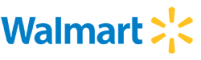New update is available. Click here to update.
Topics

# Generate All Strings

Moderate0/80
Average time to solve is 25m
0 upvote## Problem statement

You are given a string ‘S’ of length ‘N’ which consists of digits from 0 to 9 only. If the mapping of characters from a to z is like a = 1, b = 2, c = 3…. Z = 26. You need to convert the given string of digits into the string of characters using the mapping.

Example:
``````‘S’ = “112”.

Output: [aab, al, kb]

The possible ways to convert the given strings is:
aab => a = 1, a = 1, b = 2,
al => a = 1, l = 12
kb => k = 11, b = 2
Hence, the final array is: [aab, al, kb].
``````
Detailed explanation ( Input/output format, Notes, Images )
Constraints :
``````1 <= T <= 10
1 <= S.length <= 20
Sum of length of strings over all test cases <= 20

Time Limit: 1 sec
``````
Sample Input 1 :
``````2
1123
125
``````
Sample Output 1 :
``````aabc aaw alc kbc kw
abe ay le
``````
Explanation Of Sample Input 1 :
``````For the first case:
aabc => a=1, a=1, b=2, c=3
aaw => a=1, a=1, w=23
alc => a=1, l=12, c=3
kbc => k=11, b=2, c=3
kw => k=11, w=23
Sorting the strings in lexicographical order, so the final output is [aabc, aaw, alc, kbc, kw].

For the second case:
abe => a=1, b=2, e=5
le => l=12, e=5
Sorting the strings in lexicographical order, the final output is [abe le].
``````
Sample Input 2 :
``````2
721023
871121
``````
Sample Output 2 :
``````gbjbc gbjw
hgaaba hgaau hgala hgkba hgku
``````Console